# 【我和openGauss的故事】openGauss 5.0.0 数据库的索引

0收藏

### 前言

索引是一个指向表中数据的指针。一个数据库中的索引与一本书的索引目录是非常相似的。

索引可以用来提高数据库查询性能，但是不恰当的使用将导致数据库性能下降。建议仅在匹配如下某条原则时创建索引：

1. 经常执行查询的字段。
2. 在连接条件上创建索引，对于存在多字段连接的查询，建议在这些字段上建立组合索引。例如，select * from t1 join t2 on t1.a=t2.a and t1.b=t2.b，可以在t1表上的a，b字段上建立组合索引。
3. WHERE子句的过滤条件字段上（尤其是范围条件）。
4. 经常出现在ORDER BY、GROUP BY和DISTINCT后的字段。
第三章和第四章介绍索引推荐方法。

### 一、索引

#### 1.1 单列索引

单列索引是一个只基于表的一个列上创建的索引。

• 语法

``CREATE INDEX [ [schema_name.]index_name ] ON table_name  (column_name);``
1. 创建单列索引
• 先创建表

``````CREATE TABLE idx_tb
(
SM_SHIP_MODE_SK           INTEGER               NOT NULL,
SM_SHIP_MODE_ID           CHAR(16)              NOT NULL,
SM_TYPE                   CHAR(30)                      ,
SM_CODE                   CHAR(10)                      ,
SM_CARRIER                CHAR(20)                      ,
SM_CONTRACT               CHAR(20)
) ;``````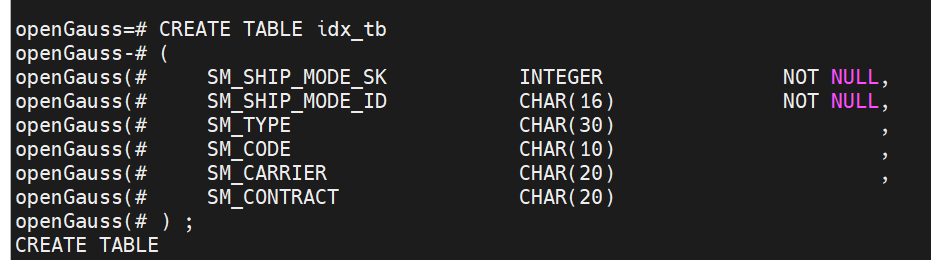• 插入数据

``````insert into idx_tb values(1,'huawei','opengauss','100','abc','abc');
insert into idx_tb values(1,'huawei1','opengauss','100','abc','abc');
insert into idx_tb values(2,'huawei2','opengauss','100','abc','abc');
insert into idx_tb values(3,'huawei3','opengauss','100','abc','abc');
insert into idx_tb values(4,'huawei4','opengauss','100','abc','abc');
insert into idx_tb values(5,'huawei5','opengauss','100','abc','abc');
insert into idx_tb values(6,'huawei6','opengauss','100','abc','abc');``````
• 在表上添加单列索引

`` CREATE INDEX idx_tb_dlsy_idx ON idx_tb(SM_SHIP_MODE_ID);``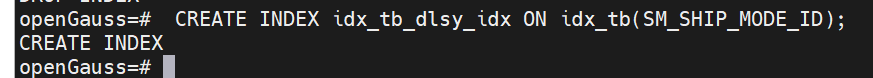• 查询

``\di idx_tb_dlsy_idx``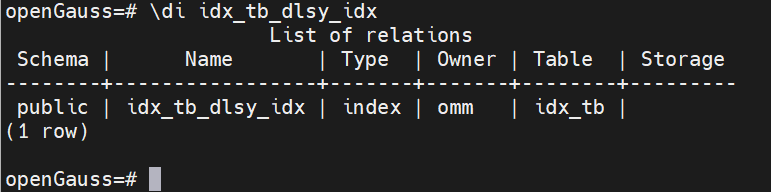• 使用单列索引

``explain select * from idx_tb where SM_SHIP_MODE_ID='huawei1';``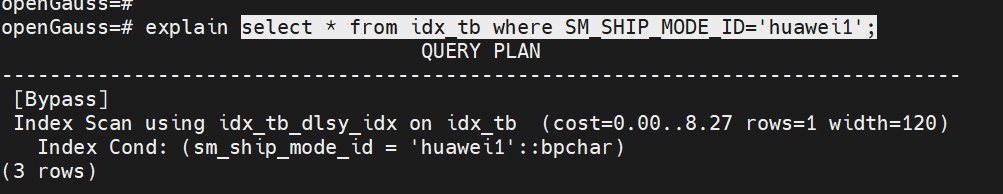#### 1.2 组合索引

组合索引是基于表的多列上创建的索引。

• 语法

``CREATE INDEX [ [schema_name.]index_name ] ON table_name  (column1_name,column2_name,...);``
• 在表上添加组合索引
在列SM_TYPE和列SM_CODE创建组合索引，索引名称：idx_tb_zhsy_idx

`` CREATE  INDEX idx_tb_zhsy_idx ON idx_tb(SM_TYPE,SM_CODE);``• 查询

``\di idx_tb_zhsy_idx``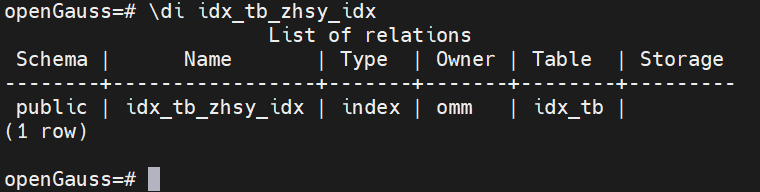• 插入数据

``````insert into idx_tb values(7,'huawei7','open','200','abc','abc');
insert into idx_tb values(8,'huawei8','gauss','300','abc','abc');
insert into idx_tb values(9,'huawei9','open100','400','abc','abc');
insert into idx_tb values(10,'huawei10','gauss100','500','abc','abc');``````
• 使用组合索引

``explain select /*+ indexonlyscan(idx_tb idx_tb_zhsy_idx) */ SM_TYPE,SM_CODE from idx_tb where SM_TYPE='gauss100' and sm_code ='200';``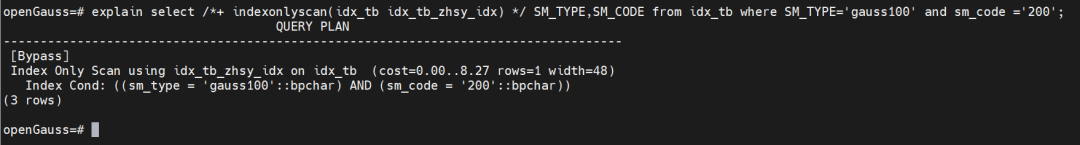#### 1.3 唯一索引

指定唯一索引的字段不允许重复值插入。

• 语法

``CREATE  UNIQUE INDEX [ [schema_name.]index_name ] ON table_name  (column_name);``
• 创建唯一索引

``CREATE UNIQUE INDEX idx_tb_uni_idx ON idx_tb(SM_SHIP_MODE_SK);``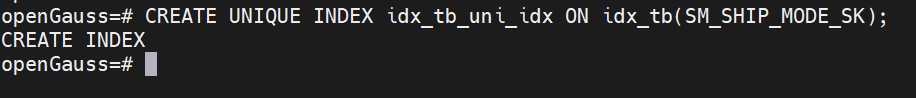• 查询

``\di idx_tb_uni_idx``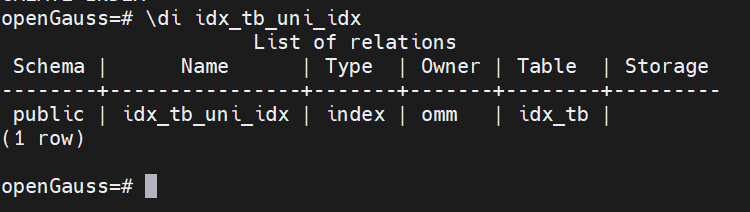• 使用唯一索引

``explain select *  from idx_tb where SM_SHIP_MODE_SK=3;``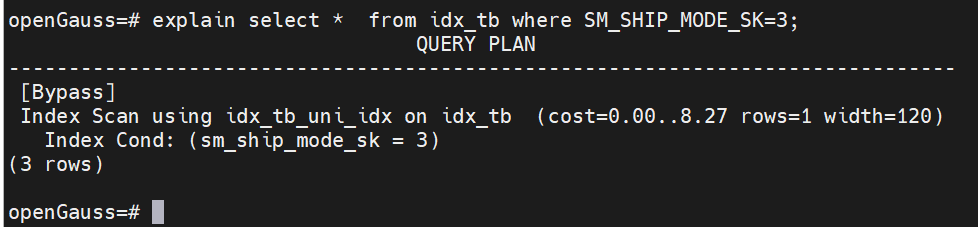#### 1.4 局部索引

在表的子集上构建索引，子集由一个条件表达式定义。

``CREATE INDEX [ [schema_name.]index_name ] ON table_name  (expression);``
• 创建局部索引

``CREATE INDEX idx_tb_jbsy_idx ON idx_tb(upper(SM_TYPE));``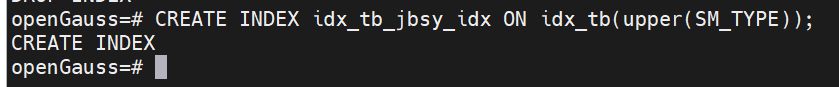• 查询

``\di idx_tb_jbsy_idx``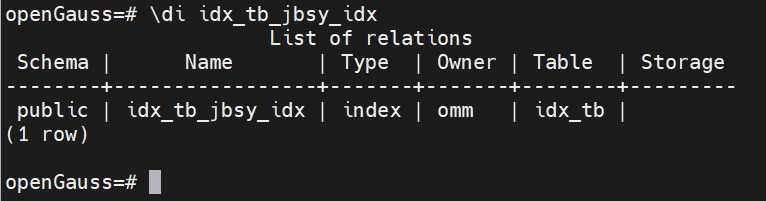• 使用局部索引

``explain analyze  select /*+ indexscan(idx_tb idx_tb_jbsy_idx) */ * from idx_tb where upper(SM_TYPE)='OPEN';``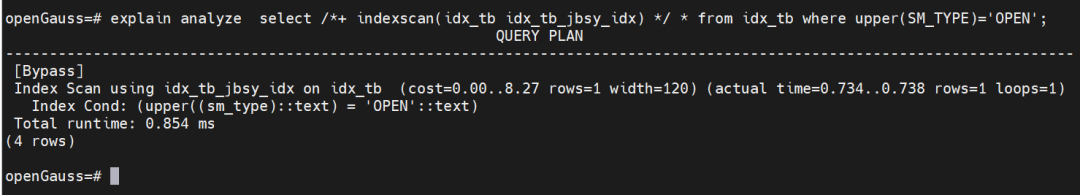#### 1.5 部分索引

部分索引是一个只包含表的一部分记录的索引，通常是该表中比其他部分数据更有用的部分。

• 语法

``CREATE INDEX [ [schema_name.]index_name ] ON table_name  (column_name) [ WHERE predicate ]``
• 创建部分索引

``CREATE  INDEX idx_tb_bfsy_idx ON idx_tb(SM_SHIP_MODE_SK) WHERE SM_SHIP_MODE_SK>4;``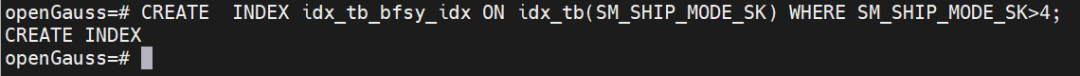• 查询

``\di idx_tb_bfsy_idx``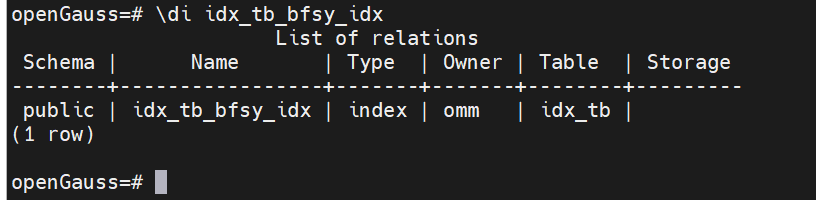• 使用部分索引

``explain select *  from idx_tb where SM_SHIP_MODE_SK=5;``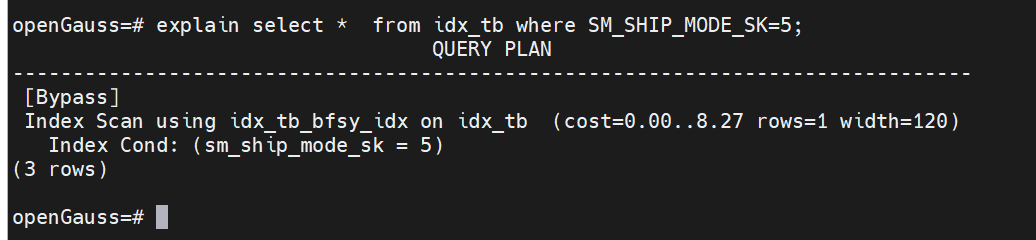#### 1.6 删除索引

• 语法

``DROP INDEX index_name;``
• 执行删除

``````DROP INDEX idx_tb_dlsy_idx;
DROP INDEX idx_tb_zhsy_idx;
DROP INDEX idx_tb_uni_idx;
DROP INDEX idx_tb_jbsy_idx;
DROP INDEX idx_tb_bfsy_idx;``````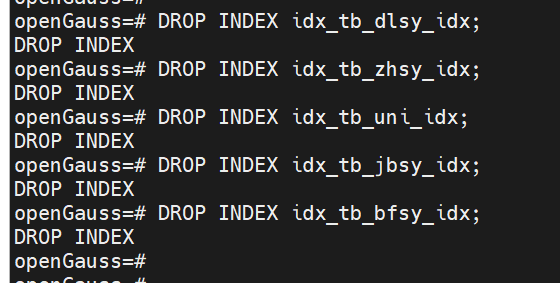### 二、 参数说明

#### 2.1 UNIQUE

创建唯一性索引，每次添加数据时检测表中是否有重复值。如果插入或更新的值会引起重复的记录时，将导致一个错误。

目前只有B-tree索引支持唯一索引。

模式的名称。

取值范围：已存在模式名。

#### 2.3 index_name

要创建的索引名，索引的模式与表相同。

取值范围：字符串，要符合标识符的命名规范。

#### 2.4 table_name

需要为其创建索引的表的名称，可以用模式修饰。

取值范围：已存在的表名。

#### 2.5 column_name

表中需要创建索引的列的名称（字段名）。

如果索引方式支持多字段索引，可以声明多个字段。全局索引最多可以声明31个字段，其他索引最多可以声明32个字段。

#### 2.6 expression

创建一个基于该表的一个或多个字段的表达式索引，通常必须写在圆括弧中。如果表达式有函数调用的形式，圆括弧可以省略。

表达式索引可用于获取对基本数据的某种变形的快速访问。比如，一个在upper(col)上的函数索引将允许WHERE upper(col) = 'JIM’子句使用索引。

在创建表达式索引时，如果表达式中包含IS NULL子句，则这种索引是无效的。此时，建议用户尝试创建一个部分索引。

#### 2.7 WHERE predicate

创建一个部分索引。部分索引是一个只包含表的一部分记录的索引，通常是该表中比其他部分数据更有用的部分。例如，有一个表，表里包含已记账和未记账的定单，未记账的定单只占表的一小部分而且这部分是最常用的部分，此时就可以通过只在未记账部分创建一个索引来改善性能。另外一个可能的用途是使用带有UNIQUE的WHERE强制一个表的某个子集的唯一性。

取值范围：predicate表达式只能引用表的字段，它可以使用所有字段，而不仅是被索引的字段。目前，子查询和聚集表达式不能出现在WHERE子句里。

### 三、虚拟索引

#### 3.1、介绍

虚拟索引功能支持用户在数据库中直接进行操作，本功能将模拟真实索引的建立，避免真实索引创建所需的时间和空间开销，用户基于虚拟索引，可通过优化器评估该索引对指定查询语句的代价影响。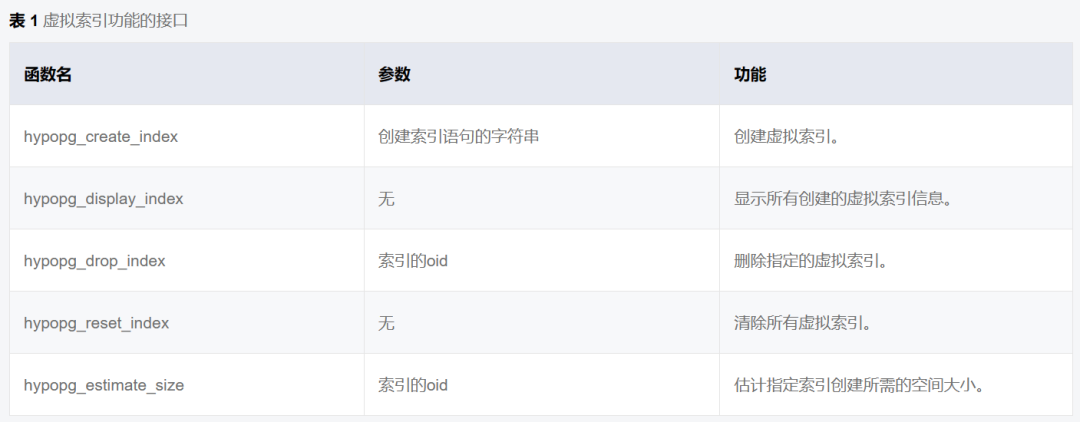#### 3.2、创建虚拟索引

使用函数hypopg_create_index创建虚拟索引

• 创建表

``CREATE TABLE hypo AS SELECT id, 'line ' || id AS val FROM generate_series(1,10000) id;``
• 创建虚拟索引

``SELECT * FROM hypopg_create_index('CREATE INDEX ON hypo (id)');``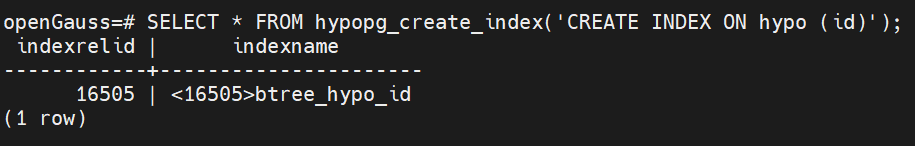• 开启enable_hypo_index前的执行计划

``EXPLAIN SELECT * FROM hypo WHERE id = 1;``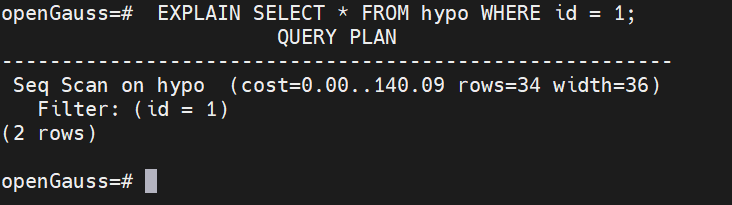#### 3.3、开启enable_hypo_index

开启GUC参数enable_hypo_index，该参数控制数据库的优化器进行EXPLAIN时是否考虑创建的虚拟索引。通过对特定的查询语句执行explain，用户可根据优化器给出的执行计划评估该索引是否能够提升该查询语句的执行效率。
enable_hypo_index的默认值为off

• 查询

``show enable_hypo_index;``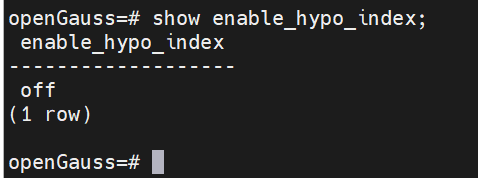• 设置enable_hypo_index值为on

``````openGauss=#  set enable_hypo_index = on;
openGauss=# show enable_hypo_index;``````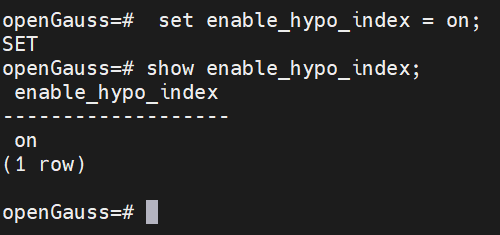#### 3.4、使用虚拟索引

• 使用虚拟索引

`` EXPLAIN SELECT * FROM hypo WHERE id = 1;``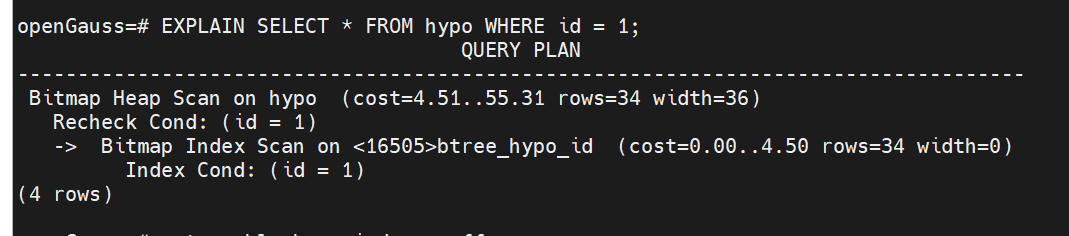• 查看真实的执行计划
可以发现实际还是全表扫描。

``EXPLAIN ANALYZE SELECT * FROM hypo WHERE id = 1;``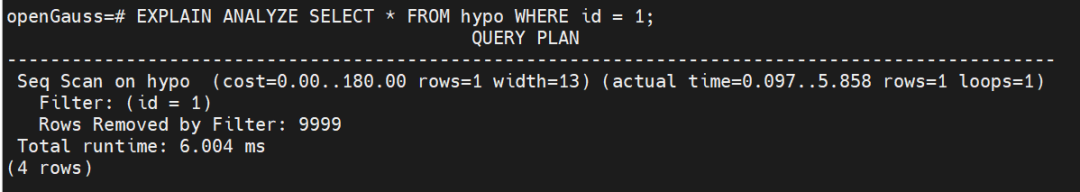#### 3.5、查询虚拟索引

``select * from hypopg_display_index();``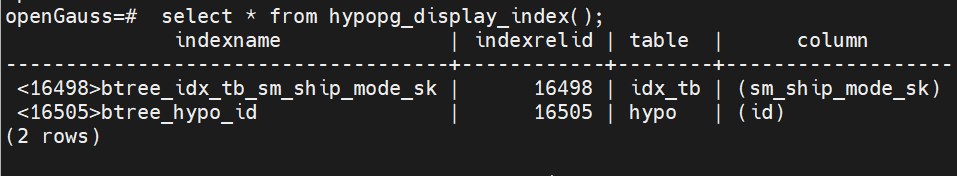#### 3.6、删除虚拟索引

• 使用函数hypopg_drop_index删除指定oid的虚拟索引

``select * from hypopg_drop_index(16505);``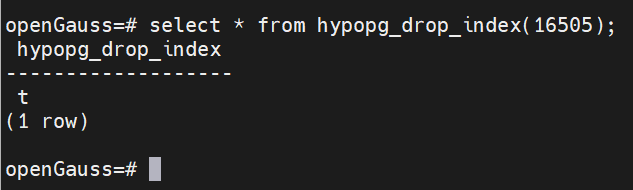• 使用函数hypopg_reset_index一次性清除所有创建的虚拟索引

``select * from hypopg_reset_index();``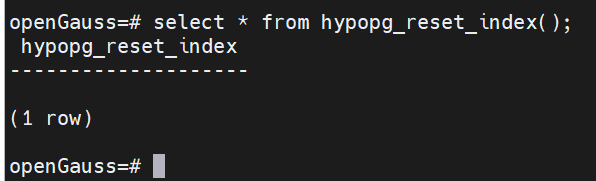#### 3.7、估算空间

• 估计指定索引创建所需的空间大小

``select * from hypopg_estimate_size(16498);``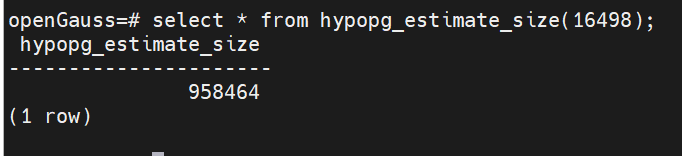### 四、单query索引推荐

#### 4.1 介绍使用上述函数，获取针对该query生成的推荐索引，推荐结果由索引的表名和列名组成。使用方法如下：

``select "table", "column" from gs_index_advise('SELECT * FROM hypo WHERE id = 1');``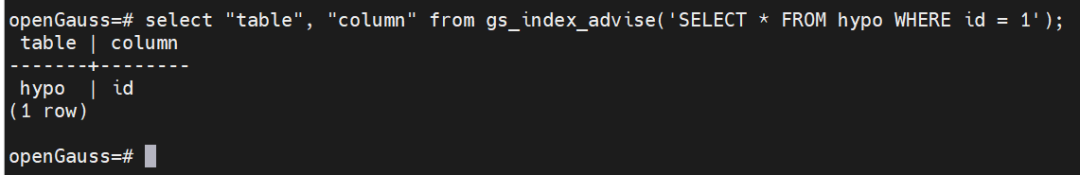#### 4.3 创建索引

``CREATE INDEX idx1 on hypo(id);``社区精华内容